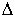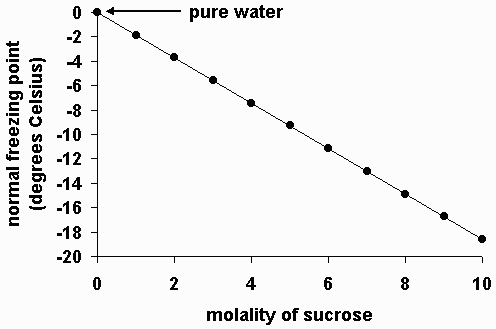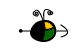Freezing Point Depression

The freezing point of a solution is less than the freezing point of the pure solvent. This means that a solution must be cooled to a lower temperature than the pure solvent in order for freezing to occur.

The freezing point of the solvent in a solution changes as the concentration of the solute in the solution changes (but it does not depend on the identity of either the solvent or the solute(s) particles (kind, size or charge) in the solution).

Non-Volatile Solutes

The freezing point of the solvent in a solution will be less than the freezing point of the pure solvent whether the solution contains a non-volatile solute or a volatile solute. However, for simplicity, only non-volatile solutes will be considered here.

Experimentally, we know that the change in freezing point of the solvent in a solution from that of the pure solvent is directly proportional to the molal concentration of the solute:T = Kfm

where:T is the change in freezing point of the solvent,
Kb is the molal freezing point depression constant, and
m is the molal concentration of the solute in the solution.

Note that the molal freezing point depression constant, Kf, has a specific value depending on the identity of the solvent.

 solvent normal freezing point, oC Kb, oC m-1 water 0.0 1.86 acetic acid 16.6 3.9 benzene 5.5 5.12 chloroform -63.5 4.68 nitrobenzene 5.67 8.1

The following graph shows the normal freezing point for water (solvent) as a function of molality in several solutions containing sucrose (a non-volatile solute). Note that the normal freezing point of water decreases as the concentration of sucrose increases.